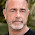## Monday, April 11, 2016

### A.D. Ellis reveals the sexy cover art of her newest book BRYAN & JASE!=•=•=•=•=•=•=•=•=•=•=•=•=•=•=•=•=•=•=•=•=•=•=•=•=•=•=•=•=

A.D. Ellis reveals the sexy cover art of her newest book, BRYAN & JASE!
It releases on April 28, 2016.

=•=•=•=•=•=•=•=•=•=•=•=•=•=•=•=•=•=•=•=•=•=•=

BLURB

Jase Rafferty grew up hiding his sexual curiosities—until he meets Bryan Keating. Bryan’s sexual preference is no secret, and being deployed overseas together allows the two men to forge a solid friendship. The sexual attraction is undeniable, but after one lust-filled week, they must go their separate ways.

A year later, a chance meeting gives them one more weekend together, even though they know Jase can’t risk his military career or his family obligations to be with Bryan.

Several years pass before Jase finally accepts the desperate longing in his heart and body. But when fear and hatred threaten to tear them apart, Jase must make a tough decision, one that he isn’t sure he and Bryan can overcome. Together, they must choose to weather the storm or say goodbye forever.

**This is a male/male romance meant for ages 18+ due to adult language and adult situations.**

=•=•=•=•=•=•=•=•=•=•=•=•=•=•=•=•=•=•=•=•=•=•=•=•=•=•=•=•=

PRESENTING

Cover Art by Andrea Michelle at Artistry in Design=•=•=•=•=•=•=•=•=•=•=•=•=•=•=•=•=•=•=•=•=•=•=

Cover Reveal Tour

Check out all the exclusive snippets from the book at –

=•=•=•=•=•=•=•=•=•=•=•=•=•=•=•=•=•=•=•=•=•=•=

=•=•=•=•=•=•=•=•=•=•=•=•=•=•=•=•=•=•=•=•=•=•=A.D. Ellis spends the majority of her days loving and wrangling two school-aged children and a husband before heading to the inner city of Indiana to teach a challenging group of alternative education students in grades third through sixth. Most days she hits the gym after school in hopes of running and lifting away the stress and headaches of the day before picking up her children and squeezing a whole day’s worth of loving and living into the too-short hours before bed. It’s no wonder Ms. Ellis lives for the slower, easier days she gets to enjoy on breaks from school.

Growing up in a small farming town in southern Indiana, A.D. is grateful to her mother for passing along the love of reading. With her nose constantly stuck in a book, Ms. Ellis became accustomed to friends and acquaintances snickering and shaking their heads at her love of reading.

A.D. never dreamed of being anything but a teacher, although there are certain times of the year when she laments her career choice. Ms. Ellis had a story idea floating in her head for about a year. After persistent prodding from a friend, A.D. put pen to paper and began writing her first story in October 2013. From that moment on, she was hooked. Taking the people and stories from her head and sharing them with readers is a scary, exhausting, rewarding, and fulfilling experience which A.D. plans to continue until there are no more stories banging around in her mind.

=•=•=•=•=•=•=•=•=•=•=•=•=•=•=•=•=•=•=•=•=•=•=

RAFFLECOPTER GIVEAWAY

Winner’s Prize: E-copy of A.D. Ellis’ Sawyer

1.1.